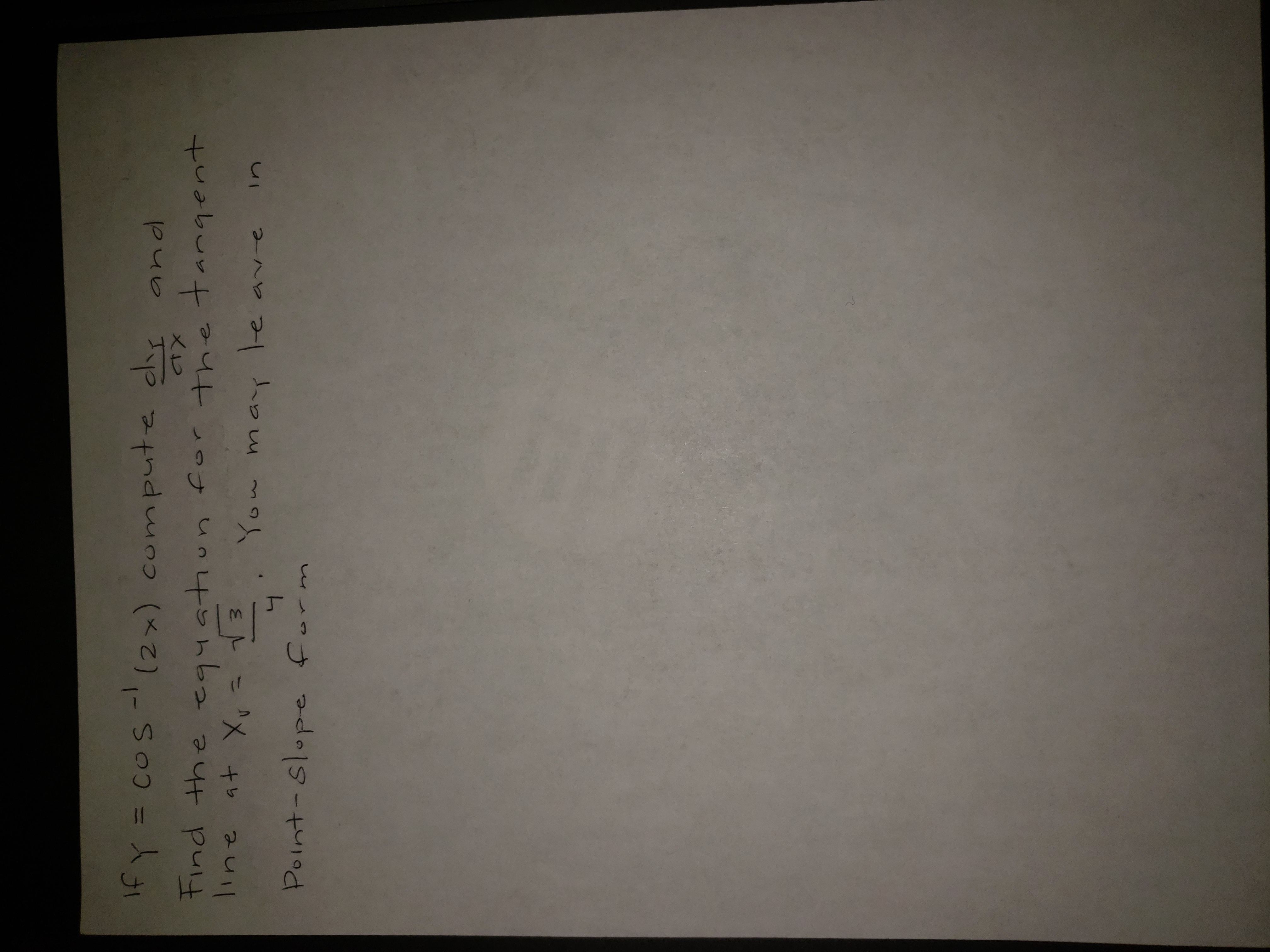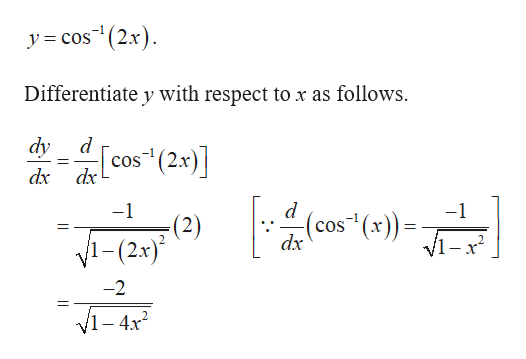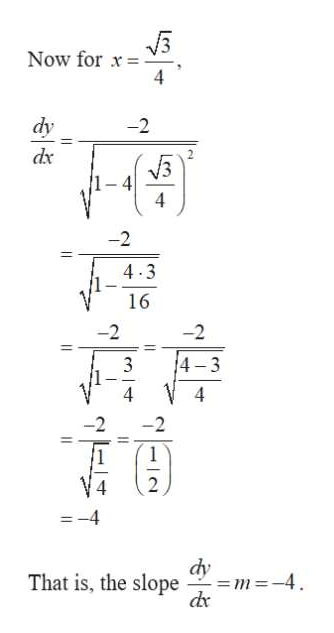# andIf y = cos-(2x) compute cliy(2x) compute dy and= COStind the equatiun for the tangentline at Xvequation forv =You may leinavePoint-slope form

Question
22 viewshelp_outlineImage Transcriptioncloseand If y = cos-(2x) compute cliy (2x) compute dy and = COS tind the equatiun for the tangent line at Xv equation for v = You may le in ave Point-slope form fullscreen
check_circle

Step 1

It is given that,help_outlineImage Transcriptionclose-1 y = cos"(2x). Differentiate y with respect to x as follows. dy d cos" (2x)] dx dx -1 -1 (cos¯(x)) = V1-x? (2) dx 1-(2x)* -2 V1 - 4x? || fullscreen
Step 2
Step 3help_outlineImage TranscriptioncloseNow for x dy -2 dx 4 -2 4.3 16 -2 -2 4-3 -4 dy = m=-4. de That is, the slope fullscreen

### Want to see the full answer?

See Solution

#### Want to see this answer and more?

Solutions are written by subject experts who are available 24/7. Questions are typically answered within 1 hour.*

See Solution
*Response times may vary by subject and question.
Tagged in

### Other